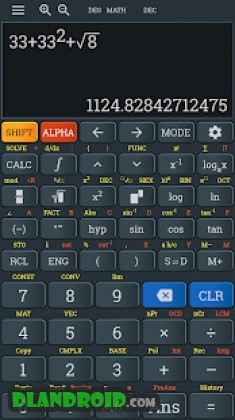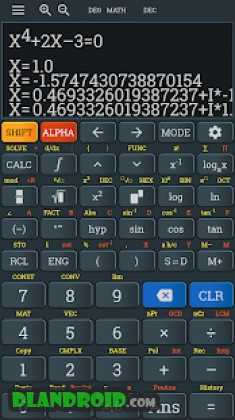Advanced calculator fx 991 es plus & 991 ms plus 4.0.2 Apk Premium latest | Download Android

Advanced calculator fx 991 es plus & 991 ms plus 4.0.2 Apk Premium latest is a Education Android app

Download last version Advanced calculator fx 991 es plus & 991 ms plus Apk Premium For Android with direct link

Advanced calculator fx 991 es plus & 991 ms plus is a Education android app made by Nhi Thien Studio that you can install on your android devices an enjoy !

Business theme

Advanced Scientific Calculator features over hundred functions and provides its user with everything they need for most mathematical calculations. The calculator’s functions include complex number calculations, matrix and vector calculations, statistics, and 40 metric conversions. Its standout feature is its 2-line natural textbook display that displays fractions, formulas, square roots and other expressions as they would in textbook. It is extremely versatile, and can be used in courses ranging from basic pre-algebra to calculus, and also has applications in physics, engineering, biology and statistics. All in one calculator, working offline, fast and powerful. A scientific calculator supports most of the features of fx 500, fx500, 570vn plus, 82ms & 82 ms, 82es & 82 es, fx 4500, 991es plus, 991ms.

Descriptions
* The Natural Display shows mathematical expressions like roots and fractions, square root, derivative, integral, matrix, … as they appear in your textbook, and this increases comprehension because results are easier to understand.
* Equation solver
* 20 pairs of values for metric conversion
* Calculation with complex numbers
* Calc key (temporary formula memory)
* 40 physical constants
* Matrix/vector calculation
* Numerical integral & differential (derivative) calculus
* Random Integers
* New equation Mode
* Function table
* Solve system of equations (two, three, four unknown variable)
* Basic arithmetic operations, powers, roots, logarithms calculator, trigonometric and hyperbolic functions
* Support conversion between rectangular and polar coordinates (POL and REC functions)
* Periodic numbers and conversion to fractions
* Generate random numbers, combinations, permutations, GCD, LCM
* Mixed fraction, Fraction, decimal, repeat decimal, polar coordinates result
* Statistic calculation, regression calculation, normal distributionAdvanced calculator fx 991 es plus & 991 ms plus ApkAdvanced calculator fx 991 es plus & 991 ms plus Apk

Whats New:

New unit converter design: now you can open unit converter from calculator or CONV dialog, and you can also transfer current expression to unit conversion calculator. With new button [ALL], the calculator supports convert to other units with single tap.
New settings: hide/show navigation bar, hide/show calculator toolbar. Button click volume setting in main screen.
Photo math: redesign scanner, improved AI.
[+-] button is replaced by percent button.

Thanks for all feedback from users.

Google Play
Download Now
Download Now
Comments
Comment